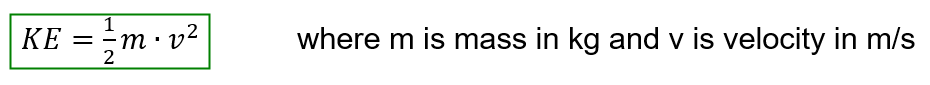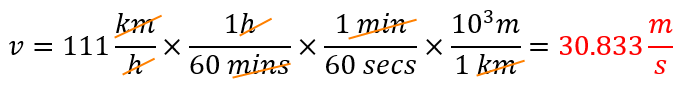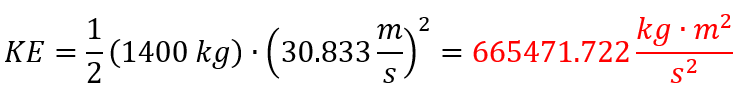# Problem: Assume that the kinetic energy of a 1400 kg car moving at 111 km/h is converted entirely into heat.What amount of water in liters could be heated from 20.0 C to 50.0 C by the car’s energy? (One calorie raises the temperature of 1 mL of water by 1 C.)

###### FREE Expert Solution

The volume of water that could be heated from 20.0°C to 50.0°C can be calculated by:

1. Determine the kinetic energy of the car

2. Determine the heat in calories from the car

3. Determine the volume of water that could be heated from the heat of the car

Step 1: Kinetic energy can be calculated from mass and velocity.Expressing the velocity in appropriate units:Calculating kinetic energy:94% (152 ratings)###### Problem Details

Assume that the kinetic energy of a 1400 kg car moving at 111 km/h is converted entirely into heat.

What amount of water in liters could be heated from 20.0 C to 50.0 C by the car’s energy? (One calorie raises the temperature of 1 mL of water by 1 C.)